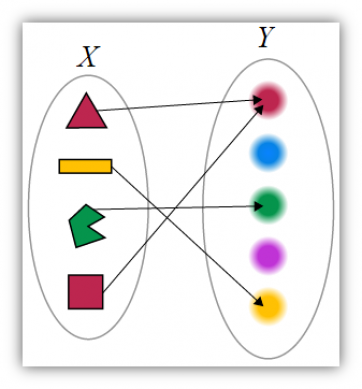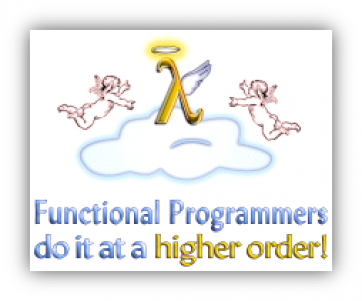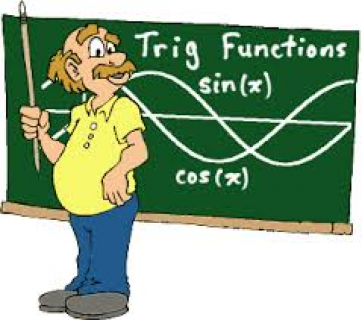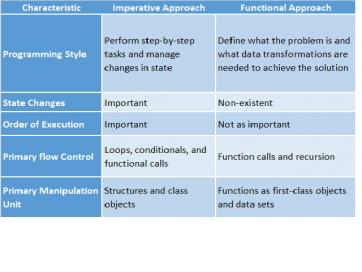# 使用 JavaScript 进行函数式编程 (一) 翻译

更新时间：2015年10月02日 13:57:40   作者：simei231, 祝青, 李中凯我要评论`var sayHello = function() { return “Hello” };`

`var person = {message: “Hello”, sayHello: function() { return “Hello” }};`

`“Hello ” + (function() { return “World” })(); //=> Hello World`

`    function hellloWorld(hello, world) { return hello + world() }`

```return “Hello”;
return function() { return “Hello”};```

### 高阶案例```[1, 2, 3].forEach(alert);
// alert 弹窗显示“1"
// alert 弹窗显示 "2"
// alert 弹窗显示 "3”```

```function splat(fun) {
return function(array) {
return fun.apply(null, array);
};
}
var addArrayElements = splat(function(x, y) { return x + y });
addArrayElements([1, 2]);
//=> 3```• 修改某个变量

• 修改数据结构

• 对外界某个变量设置字段

• 抛出例外或者弹出错误信息

### 避免修改状态```   var s = "HelloWorld";
s.toUpperCase();
//=> "HELLOWORLD"
s;
//=> "HelloWorld"```

### 不可改变状态的好处

•    避免混乱和增加程序的准确性：在复杂系统内，大多数难以理解的Bug是由于状态通过在程序中外部客户端代码修改而导致的。
•    确立“快速简洁”的多线程编程：如果多线程可以修改同一个共享值，你不得不同步的获取值。这对专家来说都是十分乏味并且易出错的编程挑战。

### 使用递归而非循环调用### 闭包的全部好处

```   function multiplier(factor) {
return function(number) {
return number * factor;
};
}
var twiceOf = multiplier(2);
console.log(twiceOf(6));
//=> 12```

### 声明式优于命令式编程

factorial(n)       = 1 if n = 1

n * factorial(n-1) if n > 1

```var imperativeFactorial = function(n) {
if(n == 1) {
return 1
} else {
product = 1;
for(i = 1; i <= n; i++) {
product *= i;
}
return product;
}
}
var declarativeFactorial = function(n) {
if(n == 1) {
return 1
} else {
return n * factorial(n - 1);
}
}```### 函数式程序员工具包

map(), filter(), 和 reduce()函数 构成了函数式程序员工具包的核心。 纯高阶函数成了函数式方法的主力。事实上，它们是纯函数和高阶函数应该仿效的典型。它们用一个函数作为输入，返回没有副作用的输出。

myArray = [1,2,3,4];

newArray = myArray.map(function(x) {return x*2});

console.log(myArray); // Output: [1,2,3,4]

console.log(newArray); // Output: [2,4,6,8]

forEach(),concat(), reverse(), sort(), every() 以及some().

### JavaScript的聚范式

JavaScript当然不是严格意义上的函数式编程语言，这也促使了对其他范式的使用：

• 命令式编程：基于详细操作描述式的编程

• 基于原型的面向对象式编程：基于原型对象及其实例的编程

• 元程序编程：操纵JavsScript执行模型的编程方式。对元程序编程的一个很好的定义描述为“编程发生在您书写代码做某事的时候，而元程序编程则发生在您书写代码导致某事的解释方式发生变化的时候。